# The discriminant statistic based on MPE-MWPE relationship and non-uniform embedding

## Vilma Petrauskiene1, Jurate Ragulskiene2, Huaxin Zhu3, Jie Wang4, Maosen Cao5

1, 2Center for Nonlinear Systems, Kaunas University of Technology, Studentu 50-146, Kaunas, LT-51368, Lithuania

3Jiangsu Zhongji Engineering Technology Research Co., Ltd., Nantong, China

4Intelligent Transportation and Intelligent Construction Engineering Research Center, Jiangsu, Dongjiao Intelligent Control Technology Group Co., Nanjing, China

5Department of Engineering Mechanics, Hohai University, Nanjing, 210098, China

5Corresponding author

Journal of Measurements in Engineering, Vol. 10, Issue 3, 2022, p. 150-163. https://doi.org/10.21595/jme.2022.22897
Received 8 June 2022; received in revised form 15 August 2022; accepted 30 August 2022; published 27 September 2022

Copyright © 2022 Vilma Petrauskiene, et al. This is an open access article distributed under the Creative Commons Attribution License, which permits unrestricted use, distribution, and reproduction in any medium, provided the original work is properly cited.
Views 14
WoS Core Citations 0
CrossRef Citations 0
Abstract.

The slope fitting line between MPE (multi-scale permutation entropy) and MWPE (multi-scale weighted permutation entropy) is recently proposed as a discriminant statistic for testing the nonlinearity of a time series. The main objective of this paper is to demonstrate that the selection of the optimal parameters of the non-uniform embedding is essential for the proposed discriminant statistic. In particular, the presented case studies indicate that the modified discriminant statistic based on non-uniform embedding can detect differences between such time series which remain indistinguishable if the original approach is used.

Keywords: time series, permutation entropy, attractor embedding.

#### 1. Introduction

Permutation entropy is a robust time series tool which provides a quantification measure of the complexity of a dynamic system by capturing the order relations between values of a time series and extracting a probability distribution of the ordinal patterns . Weighted-permutation entropy (WPE) considers the amplitude information which is ignored in the symbolization approach used in PE . PE and WPE can measure the complexity of a time series in a single scale. Multi-scale permutation entropy (MPE) and multi-scale weighted permutation entropy (MWPE) are introduced in  and do help to describe the complexity of a time series in multiple scales. It is shown in  that a linear correlation between MPE and MWPE exists in multiple scales. The slope of the linear regression between MPE and MWPE is used as a discriminant statistic to detect nonlinearity of financial time series through surrogate data analysis in .

The main objective of this paper is to employ case studies to demonstrate that the selection of optimal embedding parameters is an essential step which must be taken before this discriminant statistic (the slope of the linear regression between MPE and MWPE) can be used to characterize the complexity of a time series.

The paper is organized as follows. Short definitions of PE, WPE, MPE, WMPE, the linear regression between MPE and WMPE are given in Section 2. The selection of the optimal embedding parameters is discussed in Section 3. The comparison between the discriminant statistic and the modified discriminant statistic based on non-uniform embedding is presented in Section 4. Computational simulations with synthetic data are discussed in Section 5. Discussions on the novelty and the implications of the results are presented in Section 6. Concluding remarks are given in Section 7.

#### 2. Preliminaries

This section gives a concise description of algorithms and techniques used in .

#### 2.1. Permutation entropy and weighted permutation entropy

For a given discrete time series ${\left\{{x}_{t}\right\}}_{t=1}^{N}$, non-uniform embedding yields the trajectory matrix comprised of following row vectors [15, 11]:

(1)
${X}_{k}^{m,T}=\left\{{x}_{k},{x}_{k+{\tau }_{1}},{x}_{k+{\tau }_{1}+{\tau }_{2}},\dots ,{x}_{k+{\tau }_{1}+\dots +{\tau }_{m-1}}\right\},$

where $m$ is the dimension of the delay coordinate space; $T$ is the vector of delays; $T=\left\{{\tau }_{1},{\tau }_{2},\dots ,{\tau }_{m-1}\right\}$; $k=1,2,\dots ,n$; $n=N-\left({\tau }_{1}+\dots +{\tau }_{m-1}\right)$. Note that the trajectory matrix ${X}_{k}^{m,T}$ is constructed using overlapping observation windows (index $k$ runs successively throughout the original time series).

Permutation entropy (PE) quantifies the statistics of ordinal permutations . For example, the ordinal pattern of a sequence $\left\{8,3,5\right\}$ is $\pi =\left\{3,1,2\right\}$ because ${x}_{2}\le {x}_{3}\le {x}_{1}$.

PE of the trajectory matrix Eq. (1) is computed by the following algorithm. First, each row vector ${X}_{k}^{m,T}$ is mapped into an ordinal pattern ${\pi }_{r}^{m,T}=f\left({X}_{k}^{m,T}\right)$, $r\in \left\{1,2,\dots ,m!\right\}$; where $f$ denotes the mapping function. The relative frequency of each ordinal pattern (the probability to observe a particular ordinal pattern in the trajectory matrix) reads:

(2)
$p\left({\pi }_{l}^{m,T}\right)=\frac{\sum _{k=1}^{n}‍1\left({\pi }_{l}^{m,T}=f\left({X}_{k}^{m,T}\right)\right)}{\sum _{k=1}^{n}‍1\left(f\left({X}_{k}^{m,T}\right)\in \Pi \right)},$

where the membership function $1\left(\mathrm{\sigma }\right)$ is equal to one when the logical statement $\sigma$ is true, and equal to 0 otherwise; $\mathrm{\Pi }$ is the complete space of ordinal patterns. Finally, PE of the trajectory matrix reads:

(3)
$PE\left(m,T\right)=-{\sum }_{l=1}^{m!}‍p\left({\pi }_{l}^{m,T}\right)\mathrm{l}\mathrm{o}\mathrm{g}\left(p\left({\pi }_{l}^{m,T}\right)\right).$

Analogously, the weighted frequency of each ordinal pattern reads:

(4)
${p}_{\omega }\left({\pi }_{l}^{m,T}\right)=\frac{\sum _{k=1}^{n}‍1\left({\pi }_{l}^{m,T}=f\left({X}_{k}^{m,T}\right)\right){\omega }_{k}}{\sum _{k=1}^{n}‍1\left(f\left({X}_{k}^{m,T}\right)\in \Pi \right){\omega }_{k}},$

where ${\omega }_{k}$ is the variance of each row vector in the trajectory matrix:

(5)
${\omega }_{k}=\frac{1}{m}{\sum }_{t=1}^{m}‍{\left[{x}_{k+\left(t-1\right)T}-\overline{{X}_{k}^{m,T}}\right]}^{2},$
(6)
$\overline{{X}_{k}^{m,T}}=\frac{1}{m}{\sum }_{t=1}^{m}‍{x}_{k+\left(t-1\right)T}.$

Finally, weighted permutation entropy (WPE) of the trajectory matrix reads:

(7)
$WPE\left(m,T\right)=-{\sum }_{l=1}^{m!}‍{p}_{\omega }\left({\pi }_{l}^{m,T}\right)\mathrm{l}\mathrm{o}\mathrm{g}\left({p}_{\omega }\left({\pi }_{l}^{m,T}\right)\right).$

#### 2.2. Multi-scale permutation entropy

Multi-scale analysis is added to the calculation of PE and WPE in . Time series are analyzed at different scales by constructing consecutive coarse–grained time series by averaging a successively increasing number of data points in non-overlapping windows:

(8)
${y}_{j}^{\left(s\right)}=\frac{1}{s}{\sum }_{k=\left(j-1\right)s+1}^{js}\mathrm{‍}{x}_{k},$

where $s$ represents the scale factor and $1\le j\le \mathrm{i}\mathrm{n}\mathrm{t}\left(N/s\right)$. For a fixed $s$, PE and MPE are calculated for ${\left\{{y}_{j}^{\left(s\right)}\right\}}_{j=1}^{int\left(N/s\right)}$ resulting into the multi-scale permutation entropy (MPE) and the multi-scale weighted permutation entropy (WMPE). Note that MPE and WMPE are closely related to the Shannon information index .

#### 2.3. Linear regression between MPE and WMPE

At fixed $m$ and $T$, the computation of MPE and WMPE is performed at $s=1,2,\dots ,20$. Then, the slope of the fitting line (produced by the linear regression algorithm) between MPE and WMPE is proposed as a new discriminant statistic in .

#### 3. The selection of optimal embedding parameters

Chen and Shang  follow the recommendations from Bandt and Pompe  and use $m=3,4,\dots ,7$. However, all time delays are fixed to 1 “for the practical purposes” (as stated in ).

It has been reported in a number of studies that non-uniform embedding helps to better reveal the properties of nonlinear time series compared to uniform embedding when all time delays are equal [15, 10, 9]. Moreover, the dynamical properties of the reconstructed attractor are best revealed when the dimension of the delay coordinate space is optimal .

The role of the optimal time delay can be illustrated by the following two-dimensional example. It is well known that the selection of a particular time delay does not influence the geometric shape of the reconstructed attractor if the embedded time series is completely random [14, 11]. However, the situation is completely different if the embedded time series does represent a deterministic process (even if the signal to noise ratio is low). Fig. 1 depicts three different time series (a noise-free harmonic function, a numerical solution to the chaotic Rossler system , and a random signal) embedded at and at the optimal time delay (determined by the algorithm presented in ). It can be clearly seen that the geometric shape of the reconstructed attractor in the delay coordinate space does depend on the time delay if only the embedded time series is not a random time series.

The purpose of this comment is to demonstrate that the selection of optimal embedding parameters is an essential step which must be performed before computing the slope of the fitting line between MPE and WMPE.

#### 3.1. Optimal non-uniform embeddings of two real-world time series

Let us consider two time series nv515.dat and qbirths.dat (Fig. 2); both are standard time series available at The Time Series Data Library . Time series qbirths.dat does contain the number of daily births in Quebec (Canada) in the period between January 01, 1977 and December 31, 1990. Time series nv515.dat contains normalized tree-ring widths in dimensionless units (each tree-ring corresponds to one year vegetation period of the tree). Data are collected at Indian Pilo Garden in Nevada (USA) during the year 1980.

First of all, we determine the optimal dimension of the delay coordinate space for each individual time series. The classical FNN algorithm  yields the optimal dimension $m=5$ for both time series.

Fig. 1. The shape of the reconstructed attractor of a harmonic time series at $\tau =1$ (part A) and at $\tau =15$ (part B); the solution of the chaotic Rossler system at $\tau =1$ (part C) and at $\tau =45$ (part D); a random time series at $\tau =1$ (part E) and at $\tau =42$ (part F). All computations are performed in the two-dimensional delay coordinate plane ($m=2$). The optimal time delays are determined using the algorithm presented in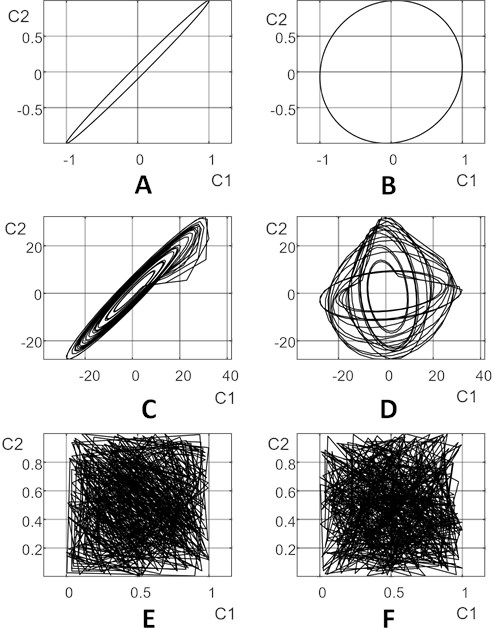Fig. 2. Time series nv515.dat (part A) and time series qbirths.dat (part B) available from TSDL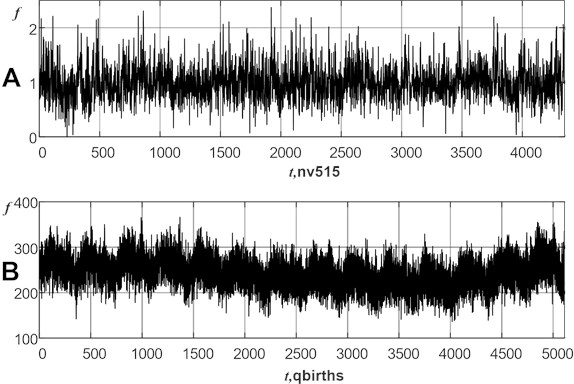Next, optimal time delays are individually determined for both time series by using the combinatorial optimization algorithm presented in . This optimization algorithm is based on the maximization of the average area of the embedded attractor in all possible planar projections . It appears that optimal time delays are: for time series nv515.dat and for time series qbirths.dat.

Fig. 3. A schematic diagram illustrating the non-uniform embedding process of time series $f\left(t\right)$ into a 5-dimensional delay coordinate space with time delays ${\tau }_{1}-{\tau }_{4}$ and coordinate axes ${C}_{1}$${C}_{5}$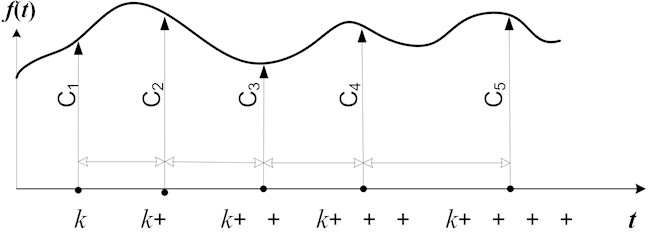All possible planar projections of the embedded attractor for time series nv515.dat at ${\tau }_{1}=1$; ${\tau }_{2}=1$; ${\tau }_{3}=1$ and ${\tau }_{4}=1$ are depicted in Fig. 3. Analogously, all possible planar projections of the embedded attractor for time series nv515.dat at ${\tau }_{1}=14$; ${\tau }_{2}=14$; ${\tau }_{3}=33$ and ${\tau }_{4}=12$ are depicted in Fig. 4.

Fig. 4. All possible planar projections of the embedded attractor of time series nv515.dat into a 5-dimensional delay coordinate space with time delays ${\tau }_{1}=1$; ${\tau }_{2}=1$; ${\tau }_{3}=1$ and ${\tau }_{4}=1$. The notations on the axes correspond to the notation used in Fig. 2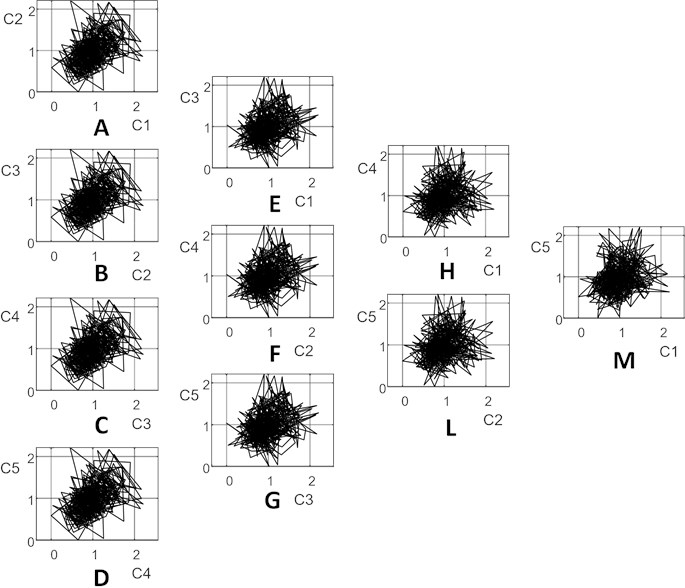A straightforward comparison between the shapes of the planar projections of the reconstructed attractors in Fig. 3 and Fig. 4 reveals that the projections of the attractor are more compressed along the hypotenuse in Fig. 3 (especially in parts A to D where time delays are equal to 1). The dynamical features of the reconstructed attractor are best revealed when the set of optimal time delays is used for its reconstruction  (Fig. 4).

Fig. 5. All possible planar projections of the embedded attractor of time series nv515.dat into a 5-dimensional delay coordinate space with optimal time delays ${\tau }_{1}=14$; ${\tau }_{2}=14$; ${\tau }_{3}=33$ and ${\tau }_{4}=12$. The notations on the axes correspond to the notation used in Fig. 2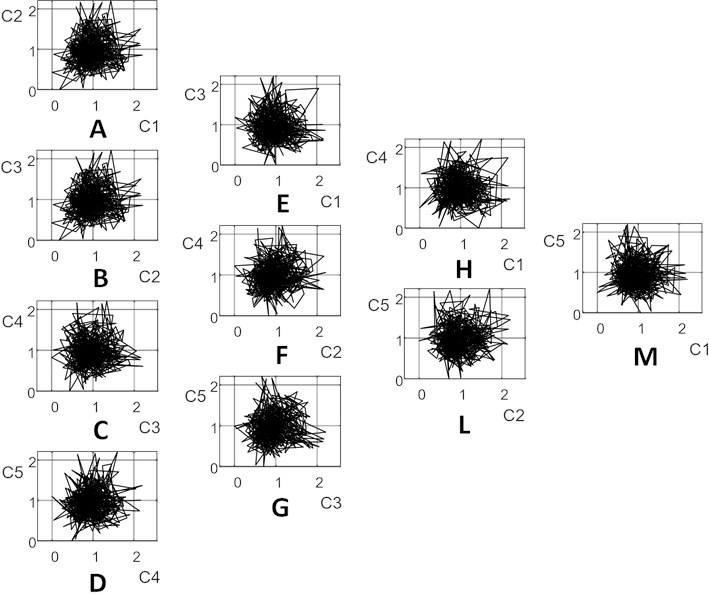#### 3.2. Considerations about the optimal embedding dimension

As mentioned previously, the FNN algorithm suggests that the optimal embedding dimension for time series nv515.dat and qbirths.dat is $m=5$. However, the embedding dimension should be determined taking into account also the length of the time series . The length of the time series $N$ should be much larger than $m!$ in order for all possible permutations to have a chance to appear . As a rule of thumb, the condition $N>5m!$ is widely adopted in the literature as a convention .

The lengths of time series nv515.dat and qbirths.dat are 4351 and 5113 correspondingly. Therefore, the condition $N>5m!$ is conveniently satisfied for both time series. However, this becomes a serious issue when coarse-grained time series are considered $\left(int\left(N/s\right)>5m!\right)$. Acceptable and unacceptable embeddings for both time series are depicted in Table 1 and Table 2. Note that optimal time delays are determined separately for each embedding dimension $m$.

It can be observed that acceptable embeddings for time series nv515.dat at $m=5$ are achieved only if the scale factor $s$ is not larger than 6 (Table 1). Analogously, acceptable embeddings for time series qbirths.dat at $m=5$ are achieved only if $s<=8$. In other words, the discriminant statistic (linear regression coefficient) between MPE and MPWE becomes an unreliable statistical parameter when the scale factor $s$ is varied between 1 and 20 (as defined in ).

Table 1. Acceptable embeddings for time series nv515.dat. The number of vectors in the trajectory matrix must be higher than $5m!$ ($m$ is the embedding dimension; $s$ is the scale factor of the coarse-grained time series; $N$ is the length of the original time series). Optimal time delays are: $T=\left\{44\right\}$ at $m=2$; at $m=3$; at $m=4$; at $m=5$; at $m=6$. Bold and italic font denote unacceptable embeddings

 $s$ $int\left(N/s\right)$ $m=$ 2 $m=$ 3 $m=$ 4 $m=$ 5 $m=$ 6 1 4351 4307 4257 4210 4278 4209 2 2176 2132 2082 2035 2103 2034 3 1450 1406 1356 1309 1377 1308 4 1088 1044 994 947 1015 946 5 870 826 776 729 797 728 6 725 681 631 584 652 583 7 622 578 528 481 549 480 8 544 500 450 403 471 402 9 483 439 389 342 410 341 10 435 391 341 294 362 293 11 396 352 302 255 323 254 12 363 319 269 222 290 221 13 335 291 241 194 262 193 14 311 267 217 170 238 169 15 290 246 196 149 217 148 16 272 228 178 131 199 130 17 256 212 162 115 183 114 18 242 198 148 101 169 100 19 229 185 135 88 156 87 20 218 174 124 77 145 76 $5m!$ 10 30 120 600 3600

Table 2. Acceptable embeddings for time series qbirths.dat. The number of vectors in the trajectory matrix must be higher than $5m!$ ($m$ is the embedding dimension; $s$ is the scale factor of the coarse-grained time series; $N$ is the length of the original time series). Optimal time delays are: $T=\left\{3\right\}$ at $m=2$; $T=\left\{3,1\right\}$ at $m=3$; $T=\left\{1,2,2\right\}$ at $m=4$; $T=\left\{2,1,2,2\right\}$ at $m=5$; $T=\left\{2,2,12,3,30\right\}$ at $m=6$. Bold and italic font denote unacceptable embeddings

 $s$ $int\left(N/s\right)$ $m=$ 2 $m=$ 3 $m=$ 4 $m=$ 5 $m=$ 6 1 5113 5110 5109 5108 5106 5064 2 2557 2554 2553 2552 2550 2508 3 1704 1701 1700 1699 1697 1655 4 1278 1275 1274 1273 1271 1229 5 1023 1020 1019 1018 1016 974 6 852 849 848 847 845 803 7 730 727 726 725 723 681 8 639 636 635 634 632 590 9 568 565 564 563 561 519 10 511 508 507 506 504 462 11 465 462 461 460 458 416 12 426 423 422 421 419 377 13 393 390 389 388 386 344 14 365 362 361 360 358 316 15 341 338 337 336 334 292 16 320 317 316 315 313 217 17 301 298 297 296 294 252 18 284 281 280 279 277 235 19 269 266 265 264 262 220 20 256 253 252 251 249 207 5$m!$ 10 30 120 600 3600

Without doubts, the optimal embedding dimension $m=5$ should be used for both time series (nv515.dat and qbirths.dat) if only the data records would be long enough. The length of the time series must be taken into account when making a decision about the acceptable embedding dimension . Table 1 and Table 2 suggest that $m=4$ would be a good compromise for both time series.

#### 4. The comparison between the discriminant statistic and the modified discriminant statistic

The algorithm presented in  is executed for both time series (nv515.dat and qbirths.dat) at $m=5$ and $T=\left\{1,1,1,1\right\}$. Relationships between MPE and MPWE are depicted in Fig. 5. The discriminant statistic (linear regression coefficient) between MPE and MPWE for time series nv515.dat at $T=\left\{1,1,1,1\right\}$ is 0.6665 (Fig. 5(a)); linear regression coefficient between MPE and MPWE for time series qbirths.dat at is 0.6639 (Fig. 5(b)). As noted previously, such computations are unreliable because the condition $int\left(N/s\right)>5m!$ does not hold true for all s (gray circles denote such MPE – MPWE points where the condition is broken).

Fig. 6. The discriminant statistic presented in  cannot determine a large difference between time series nv515.dat and qbirths.dat at $m=5$. The modified discriminant statistic (based on optimal embedding parameters) shows a large difference between time series nv515.dat and qbirths.dat at $m=4$. Linear regressions between MPE and MPWE for nv515.dat and qbirths.dat at $T=\left\{1,1,1,1\right\}$, $m=5$ are shown in parts A and B respectively. Linear regressions between MPE and MPWE for nv515.dat (at $T=\left\{46,45,50\right\}\right)$ and for qbirths.dat (at $T=\left\{1,2,2\right\}$) at $m=4$ are shown in parts C and D respectively. Gray circles denote unreliable embeddings when the condition $int\left(N/s\right)>5m!$ does not hold true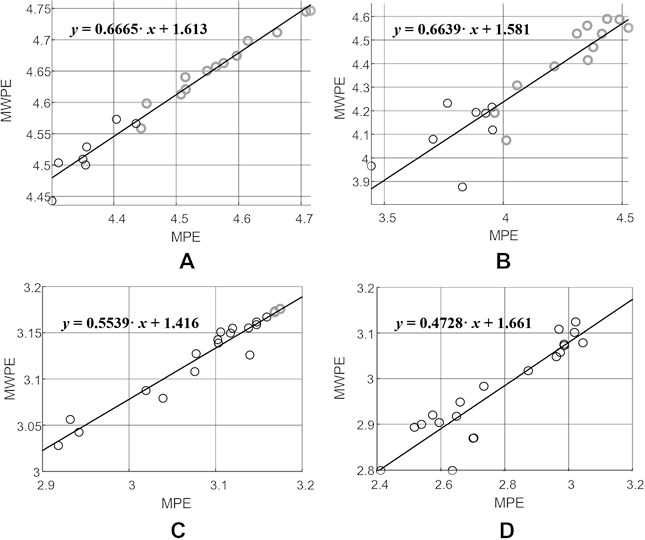Computational experiments are continued at $m=4$ and optimal time delays $T=\left\{46,45,50\right\}$ (nv515.dat) and (qbirths.dat). The discriminant statistic (linear regression coefficient) between MPE and MPWE for time series nv515.dat is 0.5539 (Fig. 5(c)); linear regression coefficient between MPE and MPWE for time series qbirths.dat is 0.4728 (Fig. 5(d)).

#### 5. Computational simulations with synthetic data

Computational simulations are continued with the chaotic Rössler system which is a paradigmatic model of chaotic dynamics :

(9)

where constants $a$, $b$, $c$ are set to 0.1, = 0.1, = 14. The Rössler time series is generated by integrating the system of equations in Eq. (9) and by selecting every tenth value of $x$ (the time step is set to 0.01). Different realizations of the discrete Gaussian random noise with zero mean are added to the synthetic Rössler time series. Optimal embedding dimensions and optimal time lags for the Rössler time series with 10 %, 50 % and 200 % noise levels are shown in Table 3 .

Table 3. Optimal embedding dimensions, optimal time lags , the discriminant statistic, the mean of the modified discriminant statistic for the Rössler time series with different noise levels

 Noise level Optimalembedding dimension Optimaltime lags The discriminant statistic $\beta$ The mean of themodified discriminantstatistic $\stackrel{^}{\beta }$ The confidence interval 10 % 6 {37, 45, 17, 38, 6} 0.9474 0.8754 [0.8521; 0.8987] 50 % 7 {36, 16, 14, 28, 35, 36} 0.9840 0.9987 [0.991; 1.0064] 200 % 8 {49, 41, 15, 36, 33, 16, 40} 0.9943 1.0238 [1.0195; 1.0281]

Linear regressions between MPE and MPWE for the Rössler time series with 10 %, 50 % and 200 % noise levels (all time lags are set to 1) are shown in Fig. 6(a, c, e). Linear regressions at non-uniform embeddings with optimal time lags are shown in Fig. 6(b, d, f). The discriminant statistic $\beta$ (reconstructed by using uniform embedding) and the modified discriminant statistic $\stackrel{^}{\beta }$ (reconstructed by using non-uniform embedding) are shown in Table 3.

Two important conclusions can be done from the results depicted in Table 6. Firstly, the modified discriminant statistic $\stackrel{^}{\beta }$ is able to determine larger differences between different time series. Secondly, the difference between $\beta$ and $\stackrel{^}{\beta }$ becomes smaller as the noise level becomes larger. This fact does correspond well to the fact that any time delays are equally good (in terms of the area occupied by the embedded attractor in the delay coordinate space) if the embedded time series is the white noise .

It is also important to evaluate the uncertainty of the modified discriminant statistic $\stackrel{^}{\beta }$. As discussed previously, the real-world time series nv515.dat and qbirths.dat are relatively short (Fig. 5). However, the chaotic Rössler time series is generated using computational algorithms without any restrictions in its length. Therefore, the slope coefficients (the modified discriminant statistic $\stackrel{^}{\beta }$) is computed ten consecutive times in ten non-overlapping observation windows. The confidence interval for $\stackrel{^}{\beta }$ using the three-sigma rule (= 0.05) is computed according to the results of repetitive computational experiments.

The confidence intervals of $\stackrel{^}{\beta }$ for the Rössler time series with 10 % noise, 50 % noise, and 200 % noise are [0.8521; 0.8987], [0.991; 1.0064], [1.0195; 1.0281] accordingly. It is interesting to observe that the discriminant statistic $\beta$ stays outside the confidence intervals of $\stackrel{^}{\beta }$ (Table 3). This is a rather natural and expected results because the chaotic Rössler time series is a stationary ergodic time series . Of course, the situation would be different if the analyzed time series would be non-stationary time series (the value of $\stackrel{^}{\beta }$ would depend on the location of the observation window).

Fig. 7. Linear regressions between MPE and MPWE for the Rössler time series with 10 %, 50 % and 200 % noise levels (all time lags are set to 1) are shown in Fig. 6 parts A, C, and E. Linear regressions for the Rössler time series with 10 %, 50 % and 200 % noise levels (optimal non-uniform time lags are set according to Table 1) are shown in Fig. 6 parts B, D, and F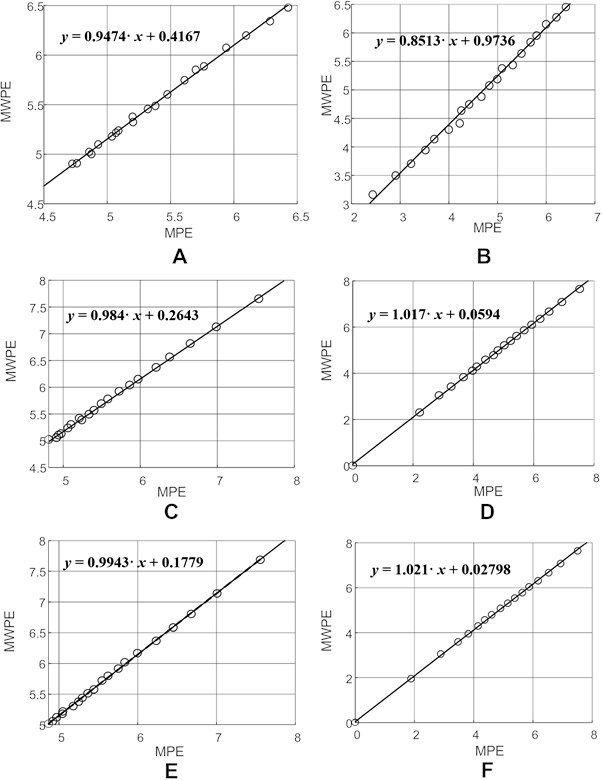#### 6. Discussions

The main objective of this paper is to demonstrate that the selection of optimal time delay(s) is an essential step in the application of discriminant statistic based on the relation between MPE and MWPE.

The objective of time delay selection methods is making components of the reconstructed vectors independent as far as possible – yet not too far, in order to keep the information about dynamic properties of the embedded time series. Moreover, optimal time lags do also result into the maximal area (in average) in all possible planar projections of the embedded attractor. Setting all time delays to 1 results into higher correlations between the components of the reconstructed vectors – what has a direct impact to the computation of MPE and MWPE. Convincing demonstrations of these effects are given in Figs. 3 and 4.

The optimization of time delays can be ignored if the time series is random, or the signal to noise ratio is very low. As mentioned previously, the selection of particular time delays is meaningless if the embedded time series does represent a random noise. Otherwise, the geometric shape of embedded attractors (and the patterns of components of the reconstructed vectors) do depend on time delays.

For example, the shapes of the planar attractors in Fig. 3 are more compressed along the diagonal if compared to Fig. 4 (this effect is especially clearly seen in parts A to D). In other words, the components of the reconstructed vectors in the trajectory matrix are more correlated in Fig. 3 than in Fig. 4.

This effect is clearly represented in the discriminant statistic based on the relation between MPE and MWPE (Fig. 5). The discriminant statistic does not show a big difference between time series nv515.dat and qbirths.dat when all time delays are set to 1. The percentage difference between the two discriminant statistic values is 0.39 % (0.6665 versus 0.6639). However, the discriminant statistic shows a much higher percentage difference when time delays are optimal: 14.64 % (0.5539 versus 0.4728).

Spearman's rank correlation coefficient is used to assess how well the relationship between MPE and MPWE can be described using a linear function. Note that a linear fit between the two variables is better for time series nv515.dat than qbirths.dat (Fig. 5). Short optimal time delays for time series qbirths.dat show that independent components of the trajectory matrix cannot be separated far away without destroying the information about dynamic properties of the embedded time series. Of course, a thorough investigation of the search space of time delays would be required to identify how strong is the global maximum in respect to other local maximums. That would help to determine how different is this time series from the random noise . Nevertheless, the fact that Spearman’s rank correlation coefficient for births.dat is lower than for nv515.dat is not astonishing. On the other hand, the comparison of Spearman’s rank correlation coefficient for Fig. 5(b) and Fig. 5(d) is meaningless because computational experiments depicted in Fig. 5(b) are based on unacceptable embeddings.

Fig. 8. Two-dimensional patterns of PE for nv515.dat time series ($m=$ 5; $T=$ 14, 14, 33, 12). Pairwise relaxation of time delays [A] results into six plane images: 14,14,i,j (part A); 14,i,33,j (part B); 14,i,j,12 (part C); i,14,33,j (part D); i,14,j,12 (part E); i,j,33,12 (part F) ); 1...50. The average of all six parts is shown in part GOptimal non-uniform time delays can be also useful for estimating basic ordinal quantifiers (PE, WPE, MPE, WMPE) – not only the proposed discriminant statistic based on the relation between MPE and WMPE. However, optimal non-uniform time delays are also useful for the extraction of much more complex ordinal features (two-dimensional patterns of PE could be a typical application discussed in detail in [16, 7].

In other words, feature extraction algorithms based on ordinal quantifiers can be conditionally classified into the following multiscale structure according to their complexity. The basic ordinal quantifiers (PE, WPE, MPE, WMPE) could be considered as the basic elements of such a multiscale feature extraction structure. The discriminant statistic (relating MPE and MWPE) can be placed in the middle of this multiscale structure. Finally, two-dimensional patterns of PE would be the most complex elements in this structure of ordinal quantifiers.

Fig. 9. Two-dimensional patterns of PE for qbirths.dat time series ($m=$ 5; $T=$ 2, 1, 2, 2). Pairwise relaxation of time delays [A] results into six plane images: 2,1,i,j (part A); 2,i,2,j (part B); 2,i,j,2 (part C); i,1,2,j (part D); i,1,j,2 (part E); i,j,2,2 (part F) ); 1...50. The average of all six parts is shown in part G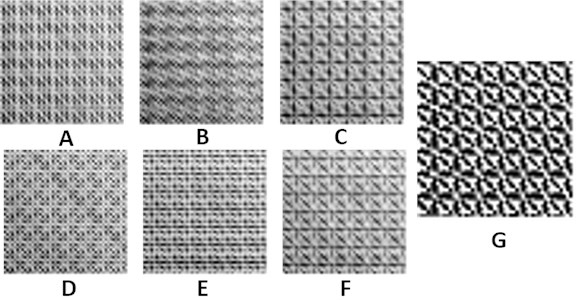Two-dimensional patterns of PE for nv515.dat and qbirths.dat time series are depicted in Fig. 7(g) and Fig. 8(g) accordingly (the resolution of those patterns is set to 50×50 pixels). As mentioned previously, the optimal embedding dimension for both time series is $m=$ 5 (the set of optimal time delays for nv515.dat is $T=$ 14, 14, 33, 12; the set of optimal time delays for qbirths.dat is $T=$ 2, 1, 2, 2). Pairwise relaxation of time delays  results into six plane images for each time series: 14,14,i,j (Fig. 7(a)); 14,i,33,j (Fig. 7(b)); 14,i,j,12 (Fig. 7(c)); i,14,33,j (Fig. 7(d)); i,14,j,12 (Fig. 7(e)); i,j,33,12 (Fig. 7(f)); 2,1,i,j (Fig. 8(a)); 2,i,2,j (Fig. 8(b)); 2,i,j,2 (Fig. 8(c)); i,1,2,j (Fig. 8(d)); i,1,j,2 (Fig. 8(e)); i,j,2,2 (Fig. 8(f)); $i$, 1,...,50. It is shown in  that deep learning based convolutional neural networks can classify the averaged patterns of PE (Fig. 7(g) and Fig. 8(g)) with an extremely high accuracy. Indeed, a naked eye can observe clear differences between Fig. 7(g) and Fig. 8(g).

The main objective of this article is to highlight the fact that non-uniform time delays play a central role not only at the top level (in terms of complexity) of ordinal quantifiers. A proper assessment of optimal time delays is crucial also in the middle level of this structure (the discriminant statistic). The latter observation is even more important because the original discriminant statistic presented in  does not pay any attention to non-uniform embeddings.

#### 7. Conclusions

Some problems regarding the use of non-overlapping windows for coarse graining, for the original MSE algorithm, are addressed in . For example, the embedding problems related to the length of the time series could be almost completely eliminated if the coarse-grained time series would be constructed using overlapping windows. However, such a construction of a coarse-grained time series would result into a simple moving average – what would induce another problems discussed in . Therefore, the construction of coarse-grained time series in this paper is performed using non-overlapping windows only.

The discriminant statistic presented in  is an interesting statistical parameter which can be used to characterize time series. However, this discriminant statistic is a result of a statistical algorithm. Therefore, it must be used with care (that statement holds true for any statistical algorithm in general). In particular, one should determine the optimal embedding dimension and the optimal set of time delays before running this algorithm for any time series.

In any case, it is always recommended to couple feature extraction algorithms based on ordinal quantifiers with the optimal parameters of non-uniform embeddings (the optimal embedding dimension and the optimal set of non-uniform time delays).

#### Acknowledgements

The authors are grateful for the International Science & Technology Cooperation Project of Jiangsu Province (BZ2022010), the Nanjing International Joint Research and Development Program (No. 202112003), and the Nantong Science and Technology Opening Cooperation Project (No. BW2021001).

1. C. Bandt and B. Pompe, “Permutation entropy: a natural complexity measure for time series,” Physical Review Letters, Vol. 88, No. 17, p. 174102, Apr. 2002, https://doi.org/10.1103/physrevlett.88.174102 [Publisher]
2. S. Chen and P. Shang, “Financial time series analysis using the relation between MPE and MWPE,” Physica A: Statistical Mechanics and its Applications, Vol. 537, p. 122716, Jan. 2020, https://doi.org/10.1016/j.physa.2019.122716 [Publisher]
3. B. Fadlallah, B. Chen, A. Keil, and J. Príncipe, “Weighted-permutation entropy: A complexity measure for time series incorporating amplitude information,” Physical Review E, Vol. 87, No. 2, p. 022911, Feb. 2013, https://doi.org/10.1103/physreve.87.022911 [Publisher]
4. A. Humeau-Heurtier, “The multiscale entropy algorithm and its variants: a review,” Entropy, Vol. 17, No. 5, pp. 3110–3123, May 2015, https://doi.org/10.3390/e17053110 [Publisher]
5. R. Hyndman and Y. Yang. “Time series data library.” pkg.yangzhuoranyang.com/tsdl. [Search CrossRef]
6. M. B. Kennel, R. Brown, and H. D. I. Abarbanel, “Determining embedding dimension for phase-space reconstruction using a geometrical construction,” Physical Review A, Vol. 45, No. 6, pp. 3403–3411, Mar. 1992, https://doi.org/10.1103/physreva.45.3403 [Publisher]
7. M. Landauskas, M. Cao, and M. Ragulskis, “Permutation entropy-based 2D feature extraction for bearing fault diagnosis,” Nonlinear Dynamics, Vol. 102, No. 3, pp. 1717–1731, Nov. 2020, https://doi.org/10.1007/s11071-020-06014-6 [Publisher]
8. M. Landauskas, Z. Navickas, A. Vainoras, and M. Ragulskis, “Weighted moving averaging revisited: an algebraic approach,” Computational and Applied Mathematics, Vol. 36, No. 4, pp. 1545–1558, Dec. 2017, https://doi.org/10.1007/s40314-016-0309-9 [Publisher]
9. K. Lukoseviciute and M. Ragulskis, “Evolutionary algorithms for the selection of time lags for time series forecasting by fuzzy inference systems,” Neurocomputing, Vol. 73, No. 10-12, pp. 2077–2088, Jun. 2010, https://doi.org/10.1016/j.neucom.2010.02.014 [Publisher]
10. Y. Manabe and B. Chakraborty, “A novel approach for estimation of optimal embedding parameters of nonlinear time series by structural learning of neural network,” Neurocomputing, Vol. 70, No. 7-9, pp. 1360–1371, Mar. 2007, https://doi.org/10.1016/j.neucom.2006.06.005 [Publisher]
11. M. Ragulskis and K. Lukoseviciute, “Non-uniform attractor embedding for time series forecasting by fuzzy inference systems,” Neurocomputing, Vol. 72, No. 10-12, pp. 2618–2626, Jun. 2009, https://doi.org/10.1016/j.neucom.2008.10.010 [Publisher]
12. O. E. Rössler, “An equation for continuous chaos,” Physics Letters A, Vol. 57, No. 5, pp. 397–398, Jul. 1976, https://doi.org/10.1016/0375-9601(76)90101-8 [Publisher]
13. M. Riedl, A. Müller, and N. Wessel, “Practical considerations of permutation entropy,” The European Physical Journal Special Topics, Vol. 222, No. 2, pp. 249–262, Jun. 2013, https://doi.org/10.1140/epjst/e2013-01862-7 [Publisher]
14. T. Sauer, J. A. Yorke, and M. Casdagli, “Embedology,” Journal of Statistical Physics, Vol. 65, No. 3-4, pp. 579–616, Nov. 1991, https://doi.org/10.1007/bf01053745 [Publisher]
15. T. Schreiber, “Interdisciplinary application of nonlinear time series methods,” Physics Reports, Vol. 308, No. 1, pp. 1–64, Jan. 1999, https://doi.org/10.1016/s0370-1573(98)00035-0 [Publisher]
16. M. Tao, K. Poskuviene, N. Alkayem, M. Cao, and M. Ragulskis, “Permutation entropy based on non-uniform embedding,” Entropy, Vol. 20, No. 8, p. 612, Aug. 2018, https://doi.org/10.3390/e20080612 [Publisher]
17. I. Timofejeva, K. Poskuviene, M. Cao, and M. Ragulskis, “Synchronization measure based on a geometric approach to attractor embedding using finite observation windows,” Complexity, Vol. 2018, pp. 1–16, Aug. 2018, https://doi.org/10.1155/2018/8259496 [Publisher]
18. Y. Yin and P. Shang, “Weighted multiscale permutation entropy of financial time series,” Nonlinear Dynamics, Vol. 78, No. 4, pp. 2921–2939, Dec. 2014, https://doi.org/10.1007/s11071-014-1636-2 [Publisher]
19. M. Zanin, L. Zunino, O. A. Rosso, and D. Papo, “Permutation entropy and its main biomedical and econophysics applications: a review,” Entropy, Vol. 14, No. 8, pp. 1553–1577, Aug. 2012, https://doi.org/10.3390/e14081553 [Publisher]